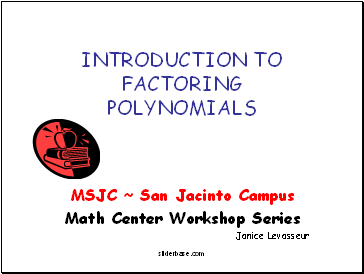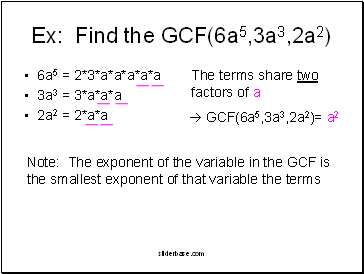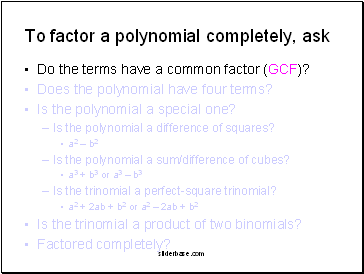# Introduction to factoring polynomialsPage 1

#### WATCH ALL SLIDES

Slide 1INTRODUCTION TO FACTORING POLYNOMIALS

MSJC ~ San Jacinto Campus

Math Center Workshop Series

Janice Levasseur

Slide 2## Definitions

Recall: Factors of a number are the numbers that divide the original number evenly.

Writing a number as a product of factors is called a factorization of the number.

The prime factorization of a number is the factorization of that number written as a product of prime numbers.

Common factors are factors that two or more numbers have in common.

The Greatest Common Factor (GCF) is the largest common factor.

Slide 3Ex: Find the GCF(24, 40).

Prime factor each number:

24

40

2

12

2

6

2

3

 24 = 2*2*2*3 = 23*3

2

20

2

10

2

5

 40 = 2*2*2*5 = 23*5

 GCF(24,40)

= 23 = 8

Slide 4The Greatest Common Factor of terms of a polynomial is the largest factor that the original terms share

Ex: What is the GCF(7x2, 3x)

7x2 = 7 * x * x

3x = 3 * x

The terms share a factor of x

 GCF(7x2, 3x) = x

Slide 5Ex: Find the GCF(6a5,3a3,2a2)

6a5 = 2*3*a*a*a*a*a

3a3 = 3*a*a*a

2a2 = 2*a*a

The terms share two factors of a

 GCF(6a5,3a3,2a2)= a2

Note: The exponent of the variable in the GCF is the smallest exponent of that variable the terms

Slide 6Definitions

To factor an expression means to write an equivalent expression that is a product

To factor a polynomial means to write the polynomial as a product of other polynomials

A factor that cannot be factored further is said to be a prime factor (prime polynomial)

A polynomial is factored completely if it is written as a product of prime polynomials

Slide 7To factor a polynomial completely, ask

Do the terms have a common factor (GCF)?

Does the polynomial have four terms?

Is the polynomial a special one?

Is the polynomial a difference of squares?

a2 – b2

Is the polynomial a sum/difference of cubes?

a3 + b3 or a3 – b3

Is the trinomial a perfect-square trinomial?

a2 + 2ab + b2 or a2 – 2ab + b2

Is the trinomial a product of two binomials?

Factored completely?

Slide 8Ex: Factor 7x2 + 3x

Go to page:
1  2  3  4  5  6  7  8  9